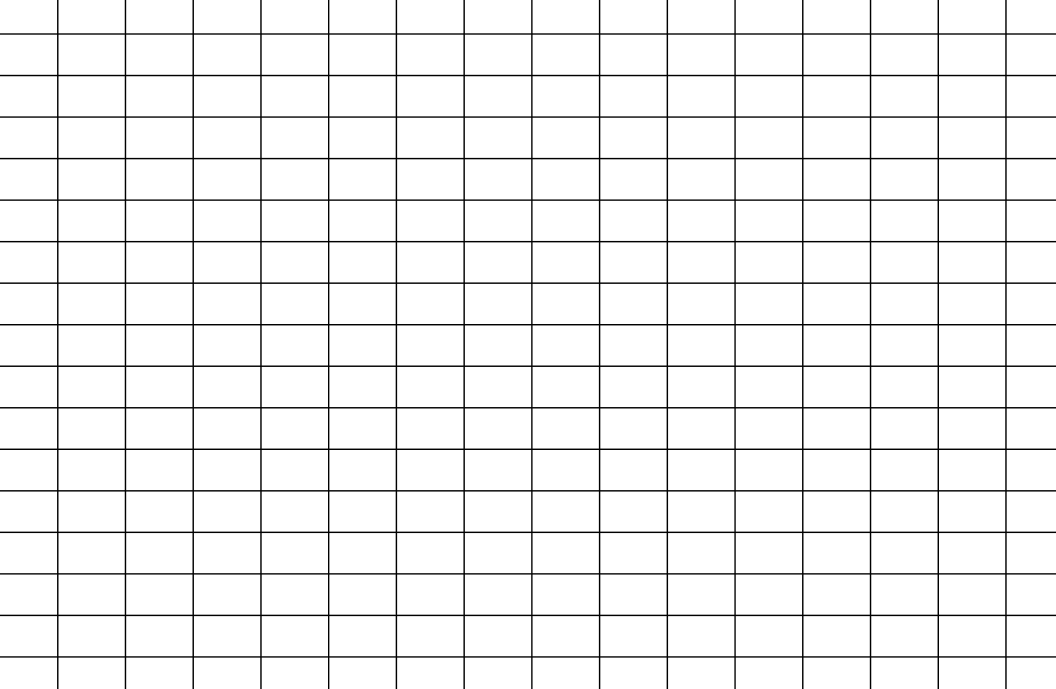# Draw a Hexagon in Java

Mehvish Ashiq Mar 14, 2022

This article will demonstrate how to draw a hexagon in Java programming. We’ll learn about arrays and objects to draw shapes on the screen.

## Use Arrays to Draw Hexagon in Java

Example code (`hexagon.java`):

``````import java.awt.Dimension;
import java.awt.Graphics;
import javax.swing.JPanel;

public class hexagon extends JPanel{

public hexagon(){
setPreferredSize(new Dimension(500, 500));
}

@Override
public void paintComponent(Graphics g){
super.paintComponent(g);
int[] xPoints = {200,300,350,300,200,150};
int[] yPoints = {350,350,250,150,150,250};
g.fillPolygon(xPoints, yPoints, xPoints.length);
}
}
``````

Example code (`drawHexagon.java`):

``````import javax.swing.JFrame;

public class drawHexagon{

public static void main(String[] args){
JFrame frame = new JFrame("Draw Hexagon");
frame.setDefaultCloseOperation(JFrame.EXIT_ON_CLOSE);
frame.pack();
frame.setLocationRelativeTo(null);
frame.setVisible(true);
}
}
``````

Output:This code example has the two classes, `hexagon.java` and `drawHexagon.java`, which has the `main` function. The `hexagon.java` extends the `JPanel` class because we override its method named `paintComponent()`.

`JPanel`, one of the `java.swing` package classes, is like a container storing various components.

Its primary task is to organize different components and layouts. You can find in-depth information about it here.

Further, we create a constructor with the `setPreferredSize()` method. It takes the object of the `Dimension` class with `width` and `height` as arguments.

Why are we using `setPreferredSize()` instead of `setBounds()` or `setSize()`? The `setSize()` and `setBounds()` can be used only when there is no layout manager being used, but we have a default layout manager.

So, we can provide some hints to a layout manager via the `setXXXXSize()` method. For instance, `setPreferredSize()`.

Then, we override a method named `paintComponent()`, which makes graphics on the screen. It takes an object from the `Graphics` class that we pass to the `paintComponent()` method of the `super` class.

We can use this object to set the color, draw the shape, etc.

After that, we create two arrays, `xPoints` and `yPoints`, having x & y coordinates. We pass these coordinates and `nPoints` to the `fillPolygon()` method to make a hexagon on the screen.

To find the exact points of a hexagon, we can use the first quadrant of the graph to get x and y points. See the following:Now, let’s talk about the `drawHexagon.java` class. We create a `JFrame` class object, which adds the hexagon to the frame using the `add()` method.

The `setDefaultCloseOperation()` gets executed as soon as a user presses the cross button (`X`) on the screen. The `setVisible(true)` method makes the window visible to the user.

The `setLocationRelativeTo(null)` method centers the computer’s screen window. Moreover, `pack()` makes a frame with the right size to carry the contents at or above their specified sizes.

## Use Object to Draw Hexagon in Java

Example code (`hexagon.java`):

``````import java.awt.Dimension;
import java.awt.Color;
import java.awt.Graphics;
import java.awt.Polygon;
import javax.swing.JPanel;

public class hexagon extends JPanel{
public hexagon(){
setPreferredSize(new Dimension(250, 250));
}

@Override
public void paintComponent(Graphics g){
super.paintComponent(g);
Polygon p = new Polygon();

for (int i = 0; i < 6; i++)
p.addPoint((int) (100 + 50 * Math.cos(i * 2 * Math.PI / 6)),
(int) (100 + 50 * Math.sin(i * 2 * Math.PI / 6)));

g.setColor(Color.RED);
g.fillPolygon(p);
}
}
``````

Main class:

``````import javax.swing.JFrame;

public class drawHexagon{
public static void main(String[] args){
JFrame frame = new JFrame("Draw Hexagon");
frame.setDefaultCloseOperation(JFrame.EXIT_ON_CLOSE);
frame.pack();
frame.setLocationRelativeTo(null);
frame.setVisible(true);
}
}
``````

Output:All functionality is the same as the previous section except for some changes in `paintComponent()` of the `hexagon.java` class.

This time, we create a `Polygon` class object to use the `addPoint()` method to add points. We set the color and fill the shape using `setColor()` and `fillPolygon()` methods.

Mehvish Ashiq is a former Java Programmer and a Data Science enthusiast who leverages her expertise to help others to learn and grow by creating interesting, useful, and reader-friendly content in Computer Programming, Data Science, and Technology.